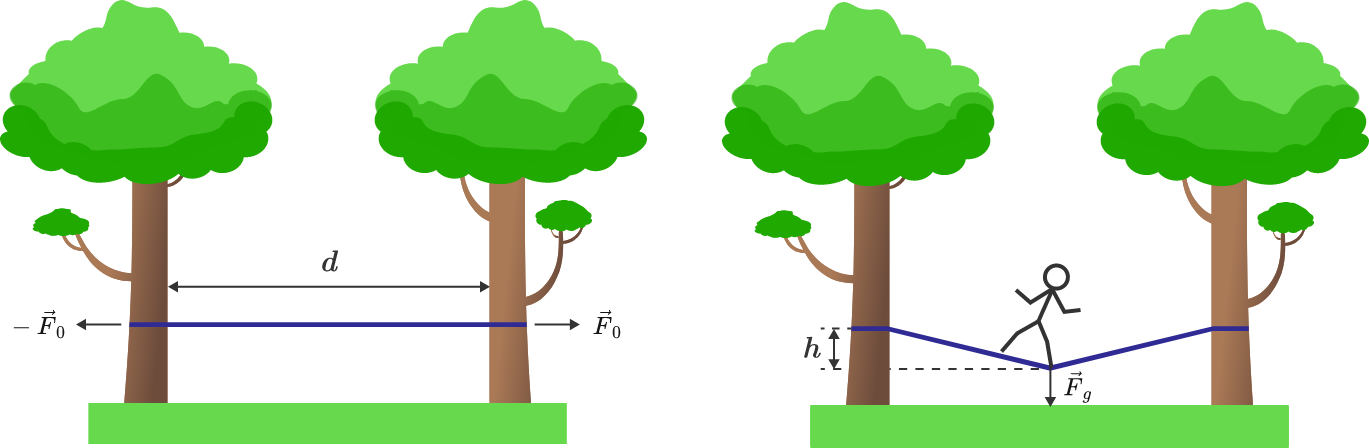# Slacklining

A slackline is stretched between two trees at a distance, $d = 10\,\text{m}$, so that a tensioning force, $F_0 = 5000 \,\text{N}$, is applied on the empty rope. A man then balances in the middle of the rope and exerts a weight force, $F_g = 750 \,\text{N}$, onto the slackline. What is the vertical deflection, $h$, of the rope?Details and Assumptions: The rope is made of an elastic material and obeys Hooke's law $F = K \frac{l - l_0}{l_0},$ where $F$ is the force along the rope, $l_0$ is the initial length, $l$ is the stretched length of the rope, and $K = 150\text{ kN}$ is the force constant.

×